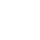# Electricity Trainer

Electricity Trainer .

We are providing our clients with qualitative Electricity Trainer. These products are designed to test basic electrical circuits. Ideal for studying electronics, these trainers teach basic of ohm’s law through simple series and parallel circuit analysis.

Object:

Experiments that can be performed:

• To study the resistances individually, as well as in series and in parallel connections
• To study the ohm’s law mathematical relation ship between three variables voltage (V), current (I) and resistance (R)
• To study the voltage and current flowing into the circuit
• To study the behavior of current when light bulbs are connected in series/parallel circuit
• To study the Kirchoff’s Law for electrical circuits
• To study the R-C circuit and find out the behavior of capacitor in a R-C network and study the phase shift due to capacitor
• To study the L-C circuit and its oscillations
• To study the characteristics of a semiconductor diode
• To study the characteristics of a transistor
• To understand the Faraday’s Law of electromagnetic induction
• To study the behavior of current when inductance is introduced in the circuit
• To study the Lenz’s Law and effect of eddy current
• To study the relay and construct a switching circuit by using relay
• To study the Oersted experiment
• To study the phenomenon of mutual induction
• To construct and study the step down transformer with the help of given coils and cores
• To construct and study the step up transformer
• To study the effects of moving I core on a step up transformer
• To convert a galvanometer into voltmeter
• To convert a galvanometer into ammeter

Specification:

• DC power supply: 5V. 200 mA
• DC power supply: 12V. 200 mA
• AC power supply: 6V, lA
• Relay: 12V
• Galvanometer: 30 -0 -30 .
• Galvanometer resistance: 80 Ohm
• Light bulbs (3 Nos.): 6V
• Potentiometers (3 Nos.): 25 Ohm. 1 W 10 K Ohm. 1 W
• Switch: 1 Pole, 2 Way Toggle Type
• Core types: E, I, U
• Main supply: 230V AC 50Hz
• Dimension: W 340 x H 110 x D 210

Accessories component box:

• Resistors - 40
• Capacitors - 04
• Transistors - 02
• Diodes - 02